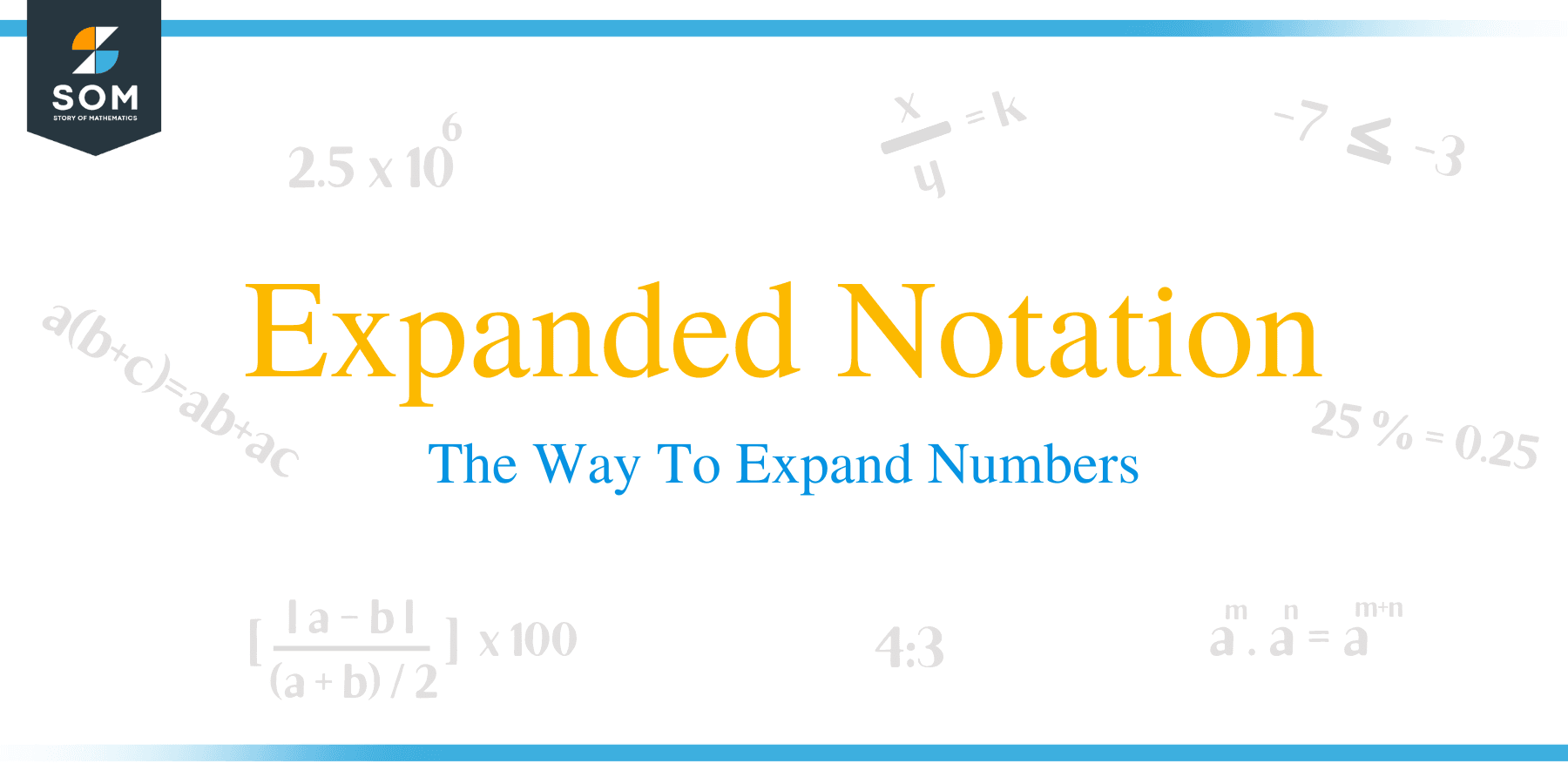# Expanded Notation – The Way to Expand Numbers

##What does Expanded Notation Mean?

Expanded notation can be defined as a way of expressing numbers by showing the value of each digit. Writing a number in expanded notation is not the same as writing in expanded form.

In expanded notation, a number is represented as the summation of each digit multiplied by its place value, whereas in expanded form, addition is only used between place value numbers. For instance:

234 in expanded form:

= 200 + 30 + 4

while 234 in expanded notation:

= (2 x 100) + (3 x 10) + (4 x 1)

The original form of the number ‘234’ is called a standard form.## How to do Expanded Notation?

To expand a particular number (from its standard form), we need to expand it into the sum of each digit multiplied by its matching place value (ones, tens, hundreds, and so on).

These methods of writing a number in expanded notation and forms are illustrated in the examples below.

Example 1

Write 4,981 in expanded form?

Solution

The number 4,981 can be written in expanded form as:

4,981 = 4,000 + 900 + 80 + 1
In this method, every number that comes after a digit is replaced with zeros. For instance, 4 and 9 in the number are represented as 4000 and 900 respectively.

Example 2

Write 15,807 in expanded form?

Solution

15,807 in expanded form is represented as:

15,807 = 10,000 + 5,000 + 800 + 7
In this example, the place value of 0 in the number is zero; therefore, the value in the tens digit is not represented because there are no tens.

Writing a number in expanded notation entails showing the place of a number in exponential powers of ten.

Example 3

Write the expanded notation of: 4,981

Solution

4,981 = (4 x 1,000) + (9 x 100) + (8 x 10) + (1 x 1)

= (4 x 10 3) + (9 x 10 2) + (8 x 10 1) + ( 1 x 10 0)

Example 4

Write 15,807 in expanded notation?

Solution

15,807 = (1 x 10,000) + (5 x 1,000) + (8 x 100) + (7 x 1)

= (1 x 10 4) + (5 x 10 3) + (8 x 10 2) + (7 x 10 0)

Example 5

Write the thousands, hundreds, tens and ones for each of the following numbers:

a. 945

945 = 9 hundreds + 4 tens + 7 ones

= 900 + 40 + 5

b. 458

458= 4 hundreds + 5 tens + 8 ones

= 400 + 50 + 8

c. 5973

5973 = 5 thousands + 9 hundreds + 7 tens + 3 ones

= 5000 + 900 + 70 + 3

d. 333

333 = 3 hundreds + 3 tens + 3 ones

= 300 + 30 + 3

e. 789

789 = 7 hundreds + 8 tens + 9 ones

= 700 + 80 + 9

### Expanded Notation with Decimals

Decimal numbers can also be written in expanded notation by using exponential powers of ten.Example 5

Write 96. 24 in expanded notation?

Solution

96.24 = 90 + 6 + 0.2 + 0.04
(9 x 10) + (6 x 1) + (3 x 10 -1) + (4 x 10 -2)

Example 6

Write the decimal number 536.072 in expanded notation.

Solution

536.072 = 500 + 30 + 6 + 0.07 + 0.002
(5 x 10 2) + (3 x 10 1) + (6 x 10 0) + (7 x 10 -2) + (2 x 10 -3)

### Practice Questions

1. Which of the following shows the completely expanded notation of the number, $90, 273$?

2. Which of the following shows the completely expanded notation of the number, $6, 587$?

3. Which of the following shows the completely expanded notation of the number, $1, 234$?

4. Which of the following shows the completely expanded notation of the number, $29, 012$?

5. Which of the following shows the completely expanded notation of the number, $49, 500$?

6. Which of the following shows the completely expanded notation of the number, $4007$?

7. Which of the following shows the standard form of $50000 + 7000 + 900 + 60 + 1$?

8. Which of the following shows the standard form of $6000 + 500 + 30 + 7$?

9. Which of the following shows the standard form of $20000 + 1000 + 200 + 70 + 9$?

10. Which of the following shows the standard form of $50000 + 7000 +10 + 8$?

11. Which of the following shows the standard form of $400000 + 80 + 8$?

12. Which of the following shows the standard form of $70000 + 7000 + 10 + 1$?

13. True or False: The number, $1749$, when written in expanded form is equal to $1000 + 700 + 40 +9$.

14. True or False: The number, $5605$, when written in expanded form is equal to $5000 + 600 + 50 + 5$.

15. True or False: The number, $43453$, when written in expanded form is equal to $4000+ 300+ 45 + 3$.

16. True or False: The number, $76125$, when written in expanded form is equal to $70000 + 6000 + 100 + 20 + 5$.

17. True or False: The number, $937032$, when written in expanded form is equal to $9 \text{ ten thousands} + 37 \text{ thousands} +3 \text{ hundreds} +2 \text{ one}$.